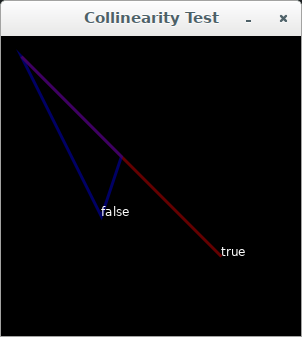You can (and should) write eloquent code in `JRubyArt` (cf vanilla `processing` and `p5*js`). Say for example you want a test to determine whether three points are collinear, you would probably look up collinear at wolfram math world. And you would see that in two dimensions given three points P1, P2 and P3 they would be collinear if their vectors x1, x2 and x3 satisfied the following equation, where x denotes the cross product.

 (x2 - x1)x(x1 - x3) = 0

We can readily translate that test into ruby (JRubyArt/propane) using `Vec2D` as our vector class (cf processing and `PVector` class), thanks to the separation of 2D and 3D functionality, and that we can simple subtract one `Vec2D` instance from another.

``````# where a, b and c are the Vec2D instances of our points
def collinear(a, b, c)
(a - b).cross(b - c).zero?
end

``````

Using the collinear test in a simple sketch

``````

def settings
size 300, 300
end

def setup
sketch_title 'Collinearity Test'
background 0
@font = create_font('Arial', 16, true)
a = Vec2D.new(20, 20)
b = Vec2D.new(120, 120)
c = Vec2D.new(220, 220)
d = Vec2D.new(100, 180)
stroke_weight 3
stroke 255, 0, 0, 100
no_fill
draw_lines a, b, c
draw_text a, b, c
stroke 0, 0, 255, 100
draw_lines a, b, d
draw_text a, b, d
end

def collinear(a, b, c)
(a - b).cross(b - c).zero?
end

def draw_lines(a, b, c)
begin_shape
vertex(a.x, a.y)
vertex(b.x, b.y)
vertex(c.x, c.y)
vertex(a.x, a.y)
end_shape CLOSE
end

def draw_text(a, b, c)
text_font(font, 12)
text("#{collinear(a, b, c)}", c.x, c.y)
end

``````Caculating the midpoint between two vectors

``````
def midpoint(a, b)
(a + b) / 2.0
end

``````